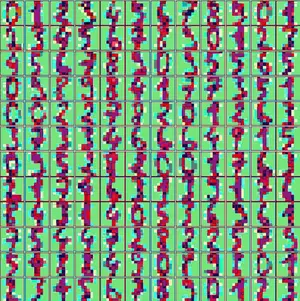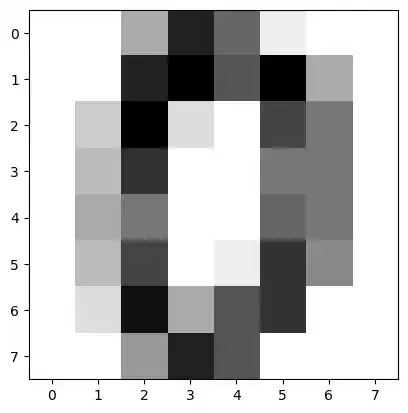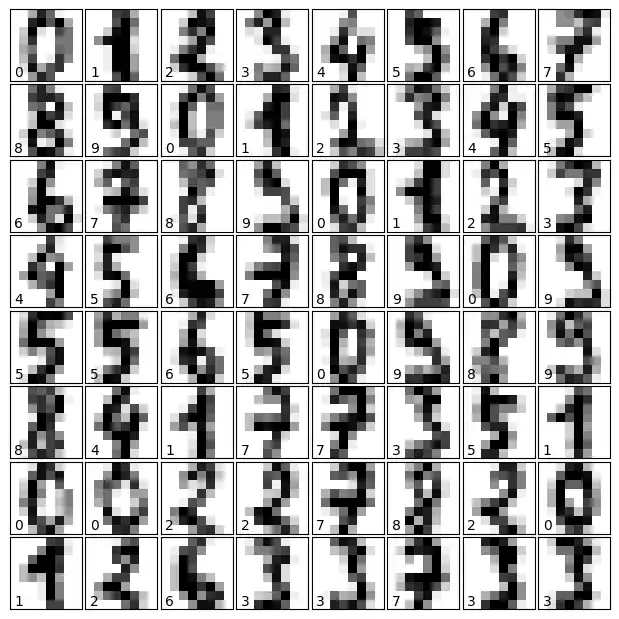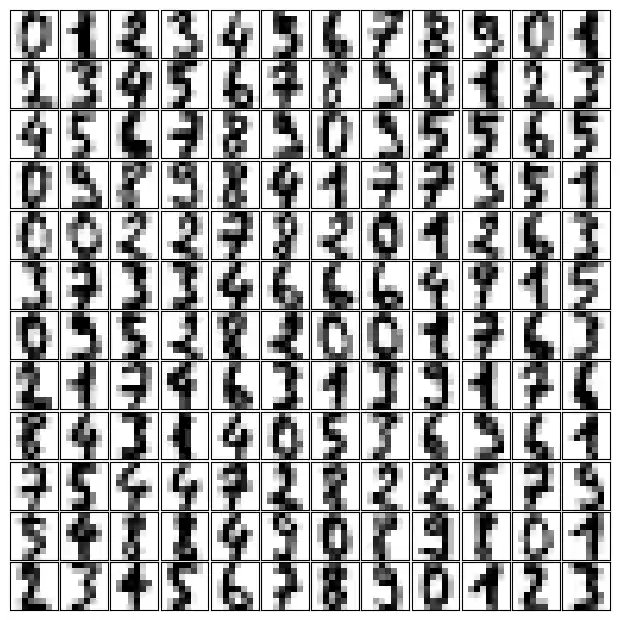# 23. A Neural Network for the Digits Dataset

## IntroductionThe Python module sklear contains a dataset with handwritten digits. It is just one of many datasets which sklearn provides, as we show in our chapter Representation and Visualization of Data. In this chapter of our Machine Learning tutorial we will demonstrate how to create a neural network for the digits dataset to recognize these digits. This example is accompanying the theoretical introductions of our previous chapters to give a practical view. You will see that hardly any Python code is needed to accomplish the actual classification and recognition task.

We will first load the digits data:

from sklearn.datasets import load_digits


We can get an overview of what is contained in the dataset with the keys method:

digits.keys()


### OUTPUT:

dict_keys(['data', 'target', 'frame', 'feature_names', 'target_names', 'images', 'DESCR'])


The digits dataset contains 1797 images and each images contains 64 features, which correspond to the pixels:

n_samples, n_features = digits.data.shape
print((n_samples, n_features))


### OUTPUT:

(1797, 64)

print(digits.data)


### OUTPUT:

[ 0.  0.  5. 13.  9.  1.  0.  0.  0.  0. 13. 15. 10. 15.  5.  0.  0.  3.
15.  2.  0. 11.  8.  0.  0.  4. 12.  0.  0.  8.  8.  0.  0.  5.  8.  0.
0.  9.  8.  0.  0.  4. 11.  0.  1. 12.  7.  0.  0.  2. 14.  5. 10. 12.
0.  0.  0.  0.  6. 13. 10.  0.  0.  0.]

print(digits.target)


### OUTPUT:

[0 1 2 ... 8 9 8]


The data is also available at digits.images. This is the raw data of the images in the form of 8 lines and 8 columns.

With "data" an image corresponds to a one-dimensional Numpy array with the length 64, and "images" representation contains 2-dimensional numpy arrays with the shape (8, 8)

print("Shape of an item: ", digits.data.shape)
print("Data type of an item: ", type(digits.data))
print("Shape of an item: ", digits.images.shape)
print("Data tpye of an item: ", type(digits.images))


### OUTPUT:

Shape of an item:  (64,)
Data type of an item:  <class 'numpy.ndarray'>
Shape of an item:  (8, 8)
Data tpye of an item:  <class 'numpy.ndarray'>


Let's visualize the data:

import matplotlib.pyplot as plt
plt.imshow(digits.images, cmap='binary')
plt.show()Let's visualize some more digits combined with their labels:

import matplotlib.pyplot as plt
# set up the figure
fig = plt.figure(figsize=(6, 6))  # figure size in inches
fig.subplots_adjust(left=0, right=1, bottom=0, top=1, hspace=0.05, wspace=0.05)

# plot the digits: each image is 8x8 pixels
for i in range(64):
ax = fig.add_subplot(8, 8, i + 1, xticks=[], yticks=[])
ax.imshow(digits.images[i], cmap=plt.cm.binary, interpolation='nearest')

# label the image with the target value
ax.text(0, 7, str(digits.target[i]))import matplotlib.pyplot as plt
# set up the figure
fig = plt.figure(figsize=(6, 6))  # figure size in inches
fig.subplots_adjust(left=0, right=1, bottom=0, top=1, hspace=0.05, wspace=0.05)

# plot the digits: each image is 8x8 pixels
for i in range(144):
ax = fig.add_subplot(12, 12, i + 1, xticks=[], yticks=[])
ax.imshow(digits.images[i], cmap=plt.cm.binary, interpolation='nearest')

# label the image with the target value
#ax.text(0, 7, str(digits.target[i]))from sklearn.model_selection import train_test_split

res = train_test_split(digits.data, digits.target,
train_size=0.8,
test_size=0.2,
random_state=1)
train_data, test_data, train_labels, test_labels = res

from sklearn.neural_network import MLPClassifier

mlp = MLPClassifier(hidden_layer_sizes=(5,),
activation='logistic',
alpha=1e-4,
solver='sgd',
tol=1e-4,
random_state=1,
learning_rate_init=.3,
verbose=True)

mlp.fit(train_data, train_labels)


### OUTPUT:

Iteration 1, loss = 2.25145782
Iteration 2, loss = 1.97730357
Iteration 3, loss = 1.66620880
Iteration 4, loss = 1.41353830
Iteration 5, loss = 1.29575643
Iteration 6, loss = 1.06663573
Iteration 7, loss = 0.95558862
Iteration 8, loss = 0.94767318
Iteration 9, loss = 0.95242867
Iteration 10, loss = 0.83577430
Iteration 11, loss = 0.74541414
Iteration 12, loss = 0.72011102
Iteration 13, loss = 0.70790928
Iteration 14, loss = 0.69425700
Iteration 15, loss = 0.74458525
Iteration 16, loss = 0.67779333
Iteration 17, loss = 0.69691846
Iteration 18, loss = 0.67844516
Iteration 19, loss = 0.68164743
Iteration 20, loss = 0.68435917
Iteration 21, loss = 0.61988051
Iteration 22, loss = 0.61362164
Iteration 23, loss = 0.56615517
Iteration 24, loss = 0.61323269
Iteration 25, loss = 0.56979209
Iteration 26, loss = 0.58189564
Iteration 27, loss = 0.50692207
Iteration 28, loss = 0.65956191
Iteration 29, loss = 0.53736180
Iteration 30, loss = 0.66437126
Iteration 31, loss = 0.56201738
Iteration 32, loss = 0.85347048
Iteration 33, loss = 0.63673358
Iteration 34, loss = 0.69769079
Iteration 35, loss = 0.62714187
Iteration 36, loss = 0.56914708
Iteration 37, loss = 1.05660379
Iteration 38, loss = 0.66966105
Training loss did not improve more than tol=0.000100 for 10 consecutive epochs. Stopping.
MLPClassifier(activation='logistic', hidden_layer_sizes=(5,),
learning_rate_init=0.3, random_state=1, solver='sgd',
verbose=True)

predictions = mlp.predict(test_data)
predictions[:25] , test_labels[:25]


### OUTPUT:

(array([1, 5, 0, 7, 1, 0, 6, 1, 5, 4, 9, 2, 7, 8, 4, 6, 9, 3, 7, 4, 7, 4,
8, 6, 0]),
array([1, 5, 0, 7, 1, 0, 6, 1, 5, 4, 9, 2, 7, 8, 4, 6, 9, 3, 7, 4, 7, 1,
8, 6, 0]))

from sklearn.metrics import accuracy_score
accuracy_score(test_labels, predictions)


### OUTPUT:

0.9611111111111111

for i in range(5, 35):
mlp = MLPClassifier(hidden_layer_sizes=(i,),
activation='logistic',
random_state=1,
alpha=1e-4,
max_iter=10000,
solver='sgd',
tol=1e-4,
learning_rate_init=.3,
verbose=False)
mlp.fit(train_data, train_labels)
predictions = mlp.predict(test_data)
acc_score = accuracy_score(test_labels, predictions)
print(i, acc_score)


### OUTPUT:

5 0.725
6 0.37222222222222223
7 0.8166666666666667
8 0.8666666666666667
9 0.8805555555555555
10 0.925
11 0.9388888888888889
12 0.9388888888888889
13 0.9388888888888889
14 0.9527777777777777
15 0.9305555555555556
16 0.95
17 0.8916666666666667
18 0.8638888888888889
19 0.9583333333333334
20 0.9638888888888889
21 0.9722222222222222
22 0.9611111111111111
23 0.9444444444444444
24 0.9583333333333334
25 0.9305555555555556
26 0.9722222222222222
27 0.9694444444444444
28 0.975
29 0.9611111111111111
30 0.9694444444444444
31 0.9694444444444444
32 0.975
33 0.95
34 0.9666666666666667


Live Python training

Enjoying this page? We offer live Python training courses covering the content of this site.

Upcoming online Courses

Enrol here